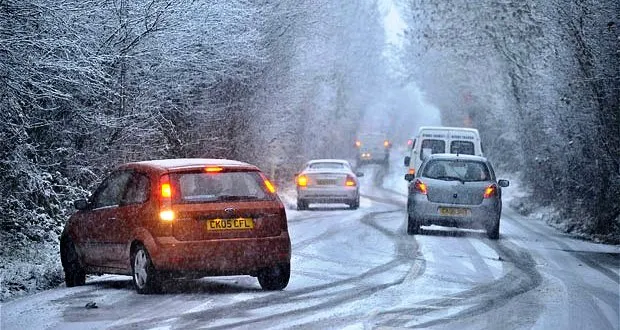# Engineering a Black Ice Detection System For Roadways

We connected a Vernier Temperature Probe and Motion Detector to an Arduino Uno to detect black ice on roads.

BeginnerFull instructions provided4,122## Things used in this project

### Hardware componentsArduino UNO & Genuino UNO
×1
 SparkFun Vernier Arduino Interface Shield
×1
 Vernier Motion Detector
×1
 Vernier Surface Temperature Sensor
×1Jumper wires (generic)
×1
×1
 SparkFun LED Array - 8x7
×1

### Software apps and online servicesArduino IDE
 codebender Used only for coding the Badgerstick

## Code

### BlackIceDetectior

Java
Upload this code onto the Arduino and when the temperature sensor detected that the ground temperature is 0°C or below, it will activate the motion detector that should light the LED when motion detector detected a decrease in distance due to the buildup of ice.
```int ledPin = 13;//plug anode in D13 and cathode in GND of LED
const int TriggerPin = 3; //trigger pin
const int EchoPin = 2;// echo pin
int ThermistorPIN =0;// Analog Pin 0
int TimeBetweenReadings = 500; // in ms

void setup() {
// put your setup code here, to run once:
pinMode(ledPin,OUTPUT);
pinMode(TriggerPin, OUTPUT);
pinMode(EchoPin, INPUT); //this is the pin that goes high when an echo is received
// initialize serial communication at 9600 bits per second:
Serial.begin(9600);
Serial.println("start");
}

void loop() {
digitalWrite(ledPin, LOW);
delay(500); //delay of half a second
// Temperature Sensors
float Time;
int Count; //reading from the A/D converter (10-bit)
float Temp; //the print below does the division first to avoid overflows (Kelvin)
Temp=Thermistor(Count);       // and  convert it to CelsiusSerial.print(Time/1000); //display in seconds, not milliseconds
}

float Thermistor(int Raw) //This function calculates temperature from ADC count
{
long Resistance;
float Resistor = 15000; //fixed resistor
float C; //Celsius
// the measured resistance of your particular fixed resistor in
// the Vernier BTA-ELV and in the SparkFun Vernier Adapter Shield
// is a precision 15K resisitor
float Temp;  // Dual-Purpose variable to save space.
Resistance=( Resistor*Raw /(1024-Raw));
Temp = log(Resistance); // Saving the Log(resistance) so not to calculate  it 4 times later
Temp = 1 / (0.00102119 + (0.000222468 * Temp) + (0.000000133342 * Temp * Temp * Temp));
C = Temp - 273.15;  // Convert Kelvin to Celsius

if(C>=0){
// Motion Detector code
long time; // clock reading in microseconds
long ReturnTime; // time it take echo to return
const float SpeedOfSound = 340; //in m/s
float Distance;// in centimeters
int val = 0;
digitalWrite(TriggerPin, LOW); //(off)
delayMicroseconds(4000);
digitalWrite(TriggerPin, HIGH); // start the ultrasound pulse (on)
time = micros(); //note time
delayMicroseconds(900); //delay during the blanking time
do
{
// if no echo, repeat loop and wait:
}
while (val == LOW) ;
ReturnTime =micros() - time;
/* The speed of sound is 340 m/s.
The ultrasound travels out and back, so to find the distance of the
object we take half of the distance traveled.*/
Distance= ReturnTime *SpeedOfSound/2/10000 ;// note convert to cm
Serial.print(ReturnTime);// print the time it took until the echo
Serial.print("\t"); // tab character
Serial.println(Distance);
delay(1000); //delay of a second

//Ice Detection

int d; //hard code distance from ground
if(C>=0 && Distance<d){
Serial.println("Black Ice Detected");
digitalWrite(ledPin, HIGH);//plug anode in D13 and cathode in GND of LED
delay(500); //delay of half a second
}
}

}
```

Java
If you have a Badgerstick like we did you may need to use the old bootloader
```#include <SparkFun_LED_8x7.h>
#include <Chaplex.h>

// Global variables
static byte led_pins[] = {2, 3, 4, 5, 6, 7, 8, 9}; // Pins for LEDs

void setup() {

// Initialize LED array
Plex.init(led_pins);

// Clear display
Plex.clear();
Plex.display();
}

void loop() {

// Scroll text 1 time
Plex.scrollText("Black Ice", 1);

// Wait 5 1/2 seconds to let the text finish scrolling
delay(5500);

}
```

## Credits

### Ankit Yande

1 project • 2 followers

### Henry Cai

1 project • 4 followers

### Shiv Rathod

1 project • 1 follower
I enjoy building things and problem solving.